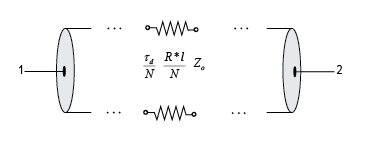# txlineDelayLossy

Create delay lossy transmission line

Since R2021a

## Description

Use the `txlineDelayLossy` object to create a delay lossy transmission line. You can also use the `txlineDelayLossy` object to model a delay lossy transmission line in an RF system using the `rfbudget` object or the RF Budget Analyzer app.## Creation

### Syntax

``dlytxline = txlineDelayLossy``
``dlytxline = txlineDelayLossy(Name,Value)``

### Description

````dlytxline = txlineDelayLossy` creates a default delay lossy transmission line object.```

example

````dlytxline = txlineDelayLossy(Name,Value)` sets Properties of the delay lossy transmission line using one or more name-value arguments. For example, `dlytxline = txlineDelayLossy('Z0',75)` creates a delay lossy transmission line with an impedance of `75` ohms.```

## Properties

expand all

Name of the delay lossy transmission line, specified as a string scalar or character vector.

Example: `'Name','DLbased'`

Example: `dlytxline.Name = 'DLbased'`

Characteristic impedance of the delay lossy transmission line, specified as a positive scalar in ohms.

Example: `'Z0',75`

Example: `dlytxline.Z0 = 75`

Physical length of the delay lossy transmission line, specified as a positive scalar in meters.

Example: `'LineLength',0.0200`

Example: `dlytxline.LineLength = 0.0200`

Time delay in the delay lossy transmission line, specified as a positive scalar in seconds.

Example: `'TimeDelay',3.7e-9`

Example: `dlytxline.TimeDelay = 3.7e-9`

Resistance value per unit of length of the delay lossy transmission line, specified as a positive scalar in ohm/meter.

Example: `'Resistance',0.3400`

Example: `dlytxline.Resistance = 0.3400`

Number of input and output ports of the delay lossy transmission line, returned as positive scalar.

Terminals of the delay lossy transmission line, returned as a cell array.

## Object Functions

 `sparameters` Calculate S-parameters for RF data, network, circuit, and matching network objects `groupdelay` Group delay of S-parameter object or RF filter object or RF Toolbox circuit object `noisefigure` Calculate noise figure of transmission lines, series RLC, and shunt RLC circuits

## Examples

collapse all

Design a coaxial cable of `0.5` meters in length as a lossy transmission line with the characteristic impedance of `48` ohms.

`dlossy = txlineDelayLossy("Z0",48,"LineLength",500e-3);`

Calculate the noise figure at `1` GHz.

`nf = noisefigure(dlossy,1e9)`
```nf = 0.0013 ```

## Algorithms

The delay lossy transmission line object calculates the S-parameters for the specified frequencies. This calculation is based on the delay line's line length, resistance, and time delay. The S-parameters are calculated using the equation given.

Here, . `ɑa` in p is the attenuation coefficient and `β` is the wave number.

The attenuation coefficient, `ɑa`, is related to the loss, `ɑ`, by

and the wave number `β` is related to the time delay, `D`, by

where `f` is the frequency range specified in the S-parameters input argument.

## Version History

Introduced in R2021a